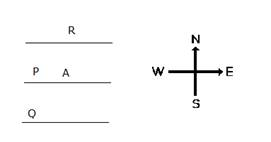## Direction Test – Practice Set 6

1. Kunal walks 10 km towards North. From there he walks 6 Km towards South. Then, he walks 3 Km towards east. How far and in which direction is he with reference to his starting point ?

a) 5 Km North

b) 5 Km South

c) 5 Km East

d) 5 Km North-East

e) none of these

d) 5 Km North-East

D

Explanation

D 5km North-east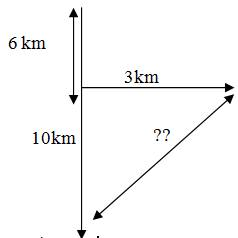√42 + 32 = √25 = 5

2. One day, Raviraj left home and cycled 20 Km southwards, turned right and cycled 10 km and turned right and cycled 20 Km and turned left and cycled 20 Km. How many kilometres will he have to cycle to reach his home straight ?

a) 50 Km

b) 30 Km

c) 40 Km

d) 60 Km

e) none of these

b) 30 Km

B

Explanation

B 30 km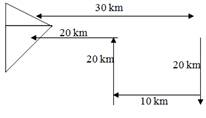D.3-5) Study the following information and answer the questions given below it:

If A is 20m South of B. D is 10m South of C.D is 20m West of E. F is 10m North of G. C is 30m east of B.F is a mid-point between D and E?

3. If D took left and walked 10m then D is in which direction with respect to B?

a) East

b) West

c) North

d) South

e) south – east

e) south – east

E

Explanation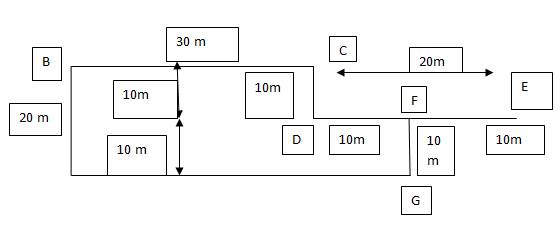south east

4. C is in which direction with respect to A?

a) South

b) South-West

c) North

d) North-East

e) East

d) North-east

D

ExplanationFrom the diagram we can easily say North-East

5. If G facing South and took right and walked a distance which 140% of distance between A and B.What is the distance between A and G now?

a) 13m

b) 11m

c) 14m

d) 12m

e) None of these

d) 12m

D

ExplanationFrom the diagram distance between A and B is 20m

140% 20m is =28m.

G took right means,

As per question Distance between A and G is = 40-28=12m

6. A boy from his home, first walks 20m in north -west direction & then 20m in south -west direction. Next, he walks 20m South-east direction. Finally he turns towards his house .In which direction is he moving?

a) North-West

b) North- East

c) South-West

d) South-East

e) South

b) North-east

B

Explanation

b)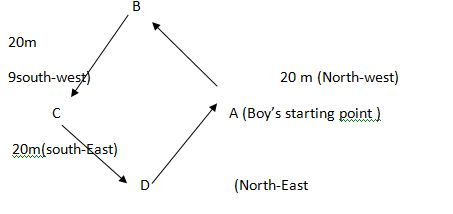7. I am facing east. I turn 100 degree in the clockwise direction and then 145 degree in the anticlockwise direction. In which direction am I facing now?

a) East

b) North-east

c) North

d) South –west

b) North-east

B

Explanation

option b)

A Man initially faces towards east in direction OA. On moving 100 degree , he faces direction OB . on further moving 145 degree anti-clockwise , he faces the direction OC makes an angle of ( 145-100) = 45 degree with OA ; so man faces North- east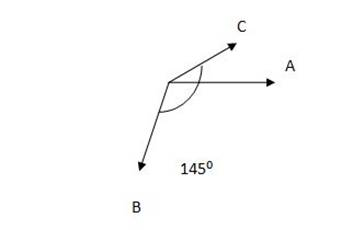D.8-9) Study the following information carefully to answer the given questions.

Point B is 12 m South of Point A. Point C is 24 m East of Point B. Point D is 8 m South of Point C. Point D is 12 m East of Point E and Point F is 8 m North of Point E. Point H is 20 m North of Point D. Point G is midpoint of the line A to H.

In which direction Point G with respect to Point B?

a) West

b) South-East

c) North-East

d) North-West

e) None of these

c) North-East

C

9. If a man has to travel to Point E from Point A (through these points by the shortest distance) , which of the following points will be pass through first?

a) Point C

b) Point D

c) Point F

d) Point B

e) None of these

d) Point B

D

10. Some boys are sitting in three rows all facing North such that A is in the middle row. P is just to the right of A but in the same row. Q is just behind of P while R is in the North of A. In which direction of R is Q?

a) South

b) South-West

c) North-East

d) South-East

e) None of these